cancel
Showing results for
Did you mean:## R script and R visuals in Power BI

Prerequisites -

R environment setup with 'maps', 'geosphere', 'data.table' packages installed.

Power BI desktop is configured for R installation.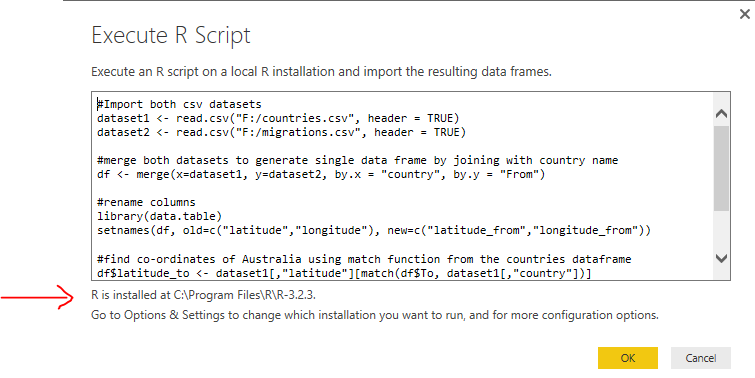I have two sample csv datasets, one containing migration statistics data from different countries to Australia over the number of years and other having list of countries with latitude and longitude co-ordinates for each.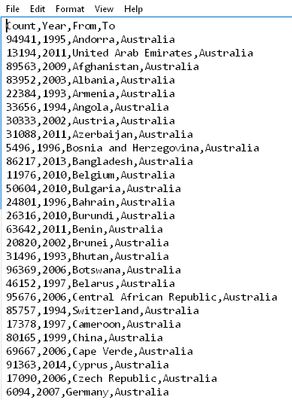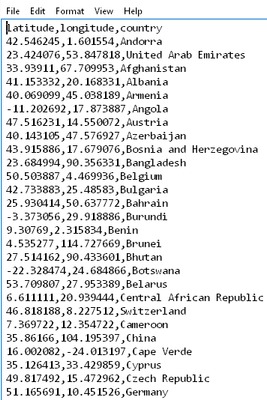To import dataset into Power BI using R, go to Get Data -> Other -> R script (Beta) and paste below code to generate data frame from above mentioned two datasets. Inside read.csv method replace ~ with appropriate folder location. Script will also prepare data frame by merging data from both csv, using match to lookup values and finally removing unwanted columns.

```#Import both csv datasets

#merge both datasets to generate single data frame by joining with country name
df <- merge(x=dataset1, y=dataset2, by.x = "country", by.y = "From")

#rename columns
library(data.table)
setnames(df, old=c("latitude","longitude"), new=c("latitude_from","longitude_from"))

#find co-ordinates of Australia using match function from the countries dataframe
df\$latitude_to <- dataset1[,"latitude"][match(df\$To, dataset1[,"country"])]
df\$longitude_to <- dataset1[,"longitude"][match(df\$To, dataset1[,"country"])]

#remove unwanted columns
df\$country <- NULL
df\$To <- NULL```

Result willl be three datasets as shown below. Click on df and Load.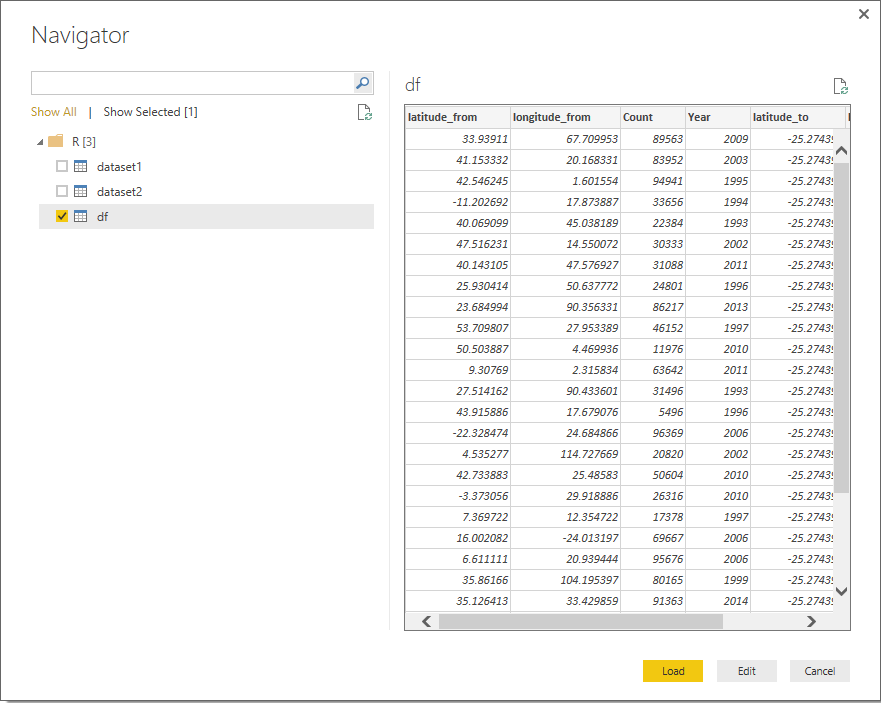Dataset will have 6 columns ready to be plotted onto map.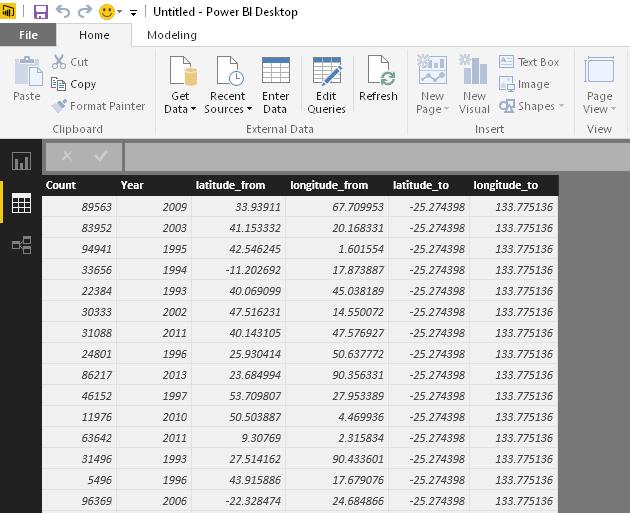Select R script visuals from visualization pane and drag all the fields into visualization pane to start scripting. Power BI at this stage will automatically create another data from the selected fields and remove duplicates for you.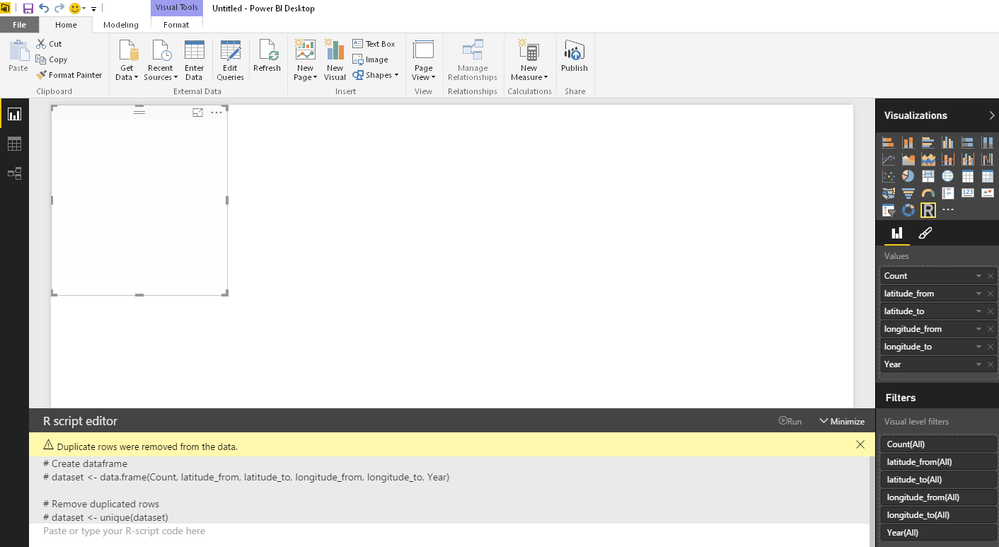Paste and run below code into R script editor to generate world map using R visual as below.

```#load maps and geosphere packages
library(maps)
library(geosphere)

#order rows in data frame by values of their count this is to ensure when lines are drawn into map
# higher count lines gets drawn on top of smaller count lines
dataset <- dataset[order(dataset\$count),]

# create basic map
map("world", fill=TRUE, bg="black", lwd=0.05)```To plot connections onto map, I will use for each loop in R to iterate over each row of the data frame and create a list of co-ordinates from and to. Then using gcIntermediate function of geosphere package which will return points on the map and using lines() function join two points returned by gcIntermediate.

```#load maps and geosphere packages
library(maps)
library(geosphere)

#order rows in data frame by values of their count this is to ensure when lines are drawn into map
# higher count lines gets drawn on top of smaller count lines
dataset <- dataset[order(dataset\$Count),]

# create basic map
map("world", col="#f2f2f2", fill=TRUE,  lwd=0.05)

#iterate over data frame, plot points on map using from and to coordinates and draw lines connecting two points
for (j in 1:length((dataset\$Year)))
{
a <- c(dataset[j,]\$longitude_from, dataset[j,]\$latitude_from)
b <- c(dataset[j,]\$longitude_to, dataset[j,]\$latitude_to)
lines(inter, col="black", lwd=0.5)
}```Let’s change some property to enhance this map. Change map colour, background and lines colour to red.

```#load maps and geosphere packages
library(maps)
library(geosphere)

#order rows in data frame by values of their count this is to ensure when lines are drawn into map
# higher count lines gets drawn on top of smaller count lines
dataset <- dataset[order(dataset\$Count),]

# create basic map
map("world", col="grey50", fill=TRUE, bg="black", lwd=0.05)

#iterate over data frame, plot points on map using from and to coordinates and draw lines connecting two points
for (j in 1:length((dataset\$Year)))
{
a <- c(dataset[j,]\$longitude_from, dataset[j,]\$latitude_from)
b <- c(dataset[j,]\$longitude_to, dataset[j,]\$latitude_to)
lines(points, col="red", lwd=0.5)
}```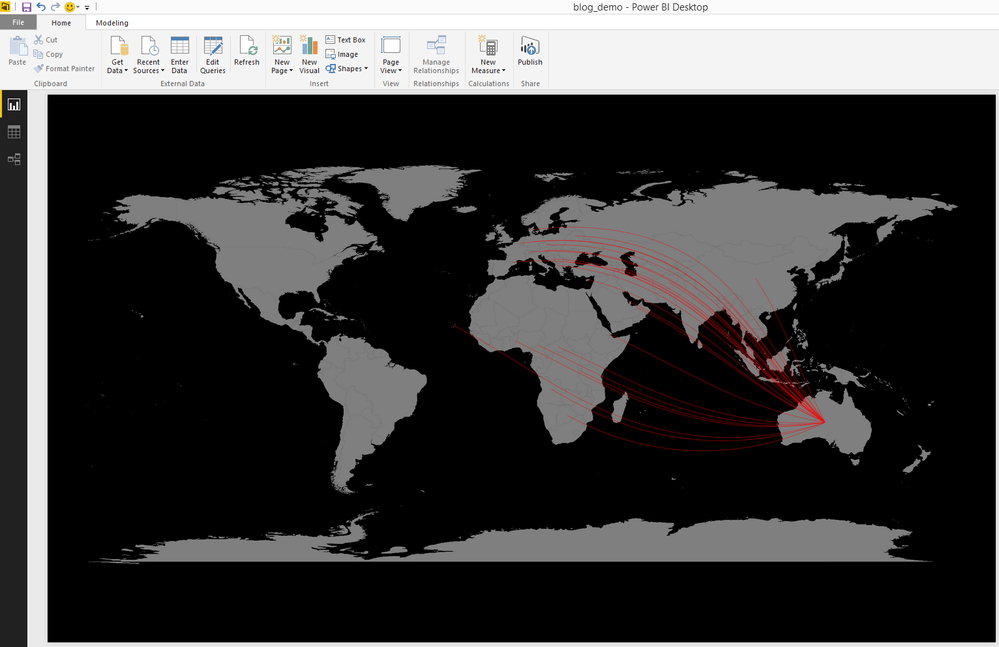It is better but still not able to distinct the proportion of population from each country. It would be nicer if there was a way to demonstrate the number of migrations by colour coding to distinct each country. Yes there is, colorRampPalette() function which returns n number of RGB values between two colours specified. Next before applying colours vector to lines calculate the colour based on number of count current row has compared to maximum count in for loop. Then apply that to lines() function.

```#load maps and geosphere packages
library(maps)
library(geosphere)

#order rows in data frame by values of their count this is to ensure when lines are drawn into map
# higher count lines gets drawn on top of smaller count lines
dataset <- dataset[order(dataset\$Count),]

# create basic map
map("world", col="grey50", fill=TRUE, bg="black", lwd=0.05)

#get max of count column
maxcnt <- max(dataset\$Count)

#getting list of colours between two colours
colors <- colorRampPalette(c("orange", "yellow"))(25)

#iterate over data frame, plot points on map using from and to coordinates and draw lines connecting two points
for (j in 1:length((dataset\$Year)))
{
a <- c(dataset[j,]\$longitude_from, dataset[j,]\$latitude_from)
b <- c(dataset[j,]\$longitude_to, dataset[j,]\$latitude_to)
colindex <- round( (dataset[j,]\$Count / maxcnt) * length(colors) )
lines(points, col=colors[colindex], lwd=0.5)
}```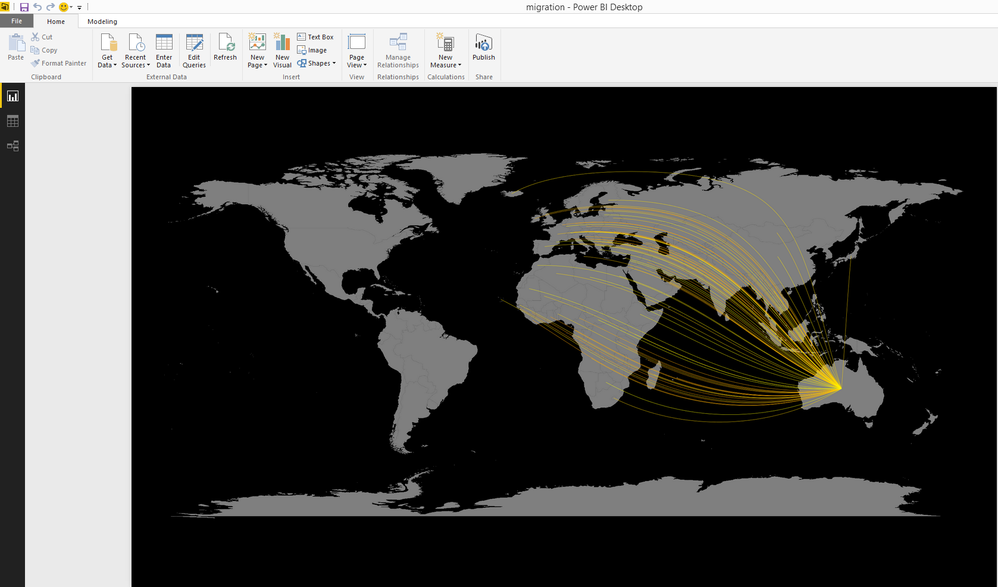It is clear to distinct that density around Asian countries is more than that of African countries and so migration count has been higher for former than the latter.